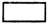# PSEB 4th Class Maths Solutions Chapter 2 Fundamental Operations on Numbers Ex 2.5

Punjab State Board PSEB 4th Class Maths Book Solutions Chapter 2 Fundamental Operations on Numbers Ex 2.5 Textbook Exercise Questions and Answers.

## PSEB Solutions for Class 4 Maths Chapter 2 Fundamental Operations on Numbers Ex 2.5

1. Fill in the blanks :

Question 1.
4 × 1 =Solution:
4

Question 2.
5 × 10 =Solution:
20Question 3.
6 × 100 =Solution:
600

Question 4.
190 × 0 =Solution:
0

Question 5.
19 ×= 1900
Solution:
100

Question 6.× 100 = 1600
Solution:
16Question 7.× 791 = 0
Solution:
0

Question 8.× 9 = 9 × 8
Solution:
8

Question 9.
4 × 10 =Solution:
40

Question 10.
7 × 100 =Solution:
700

Question 11.
9 × 1000 =Solution:
9000Question 12.
10 × 1000 =Solution:
10000

Question 13.
15 ×= 150
Solution:
10

Question 14.× 10 = 760
Solution:
76

Question 15.
798 ×= 798.
Solution:
1